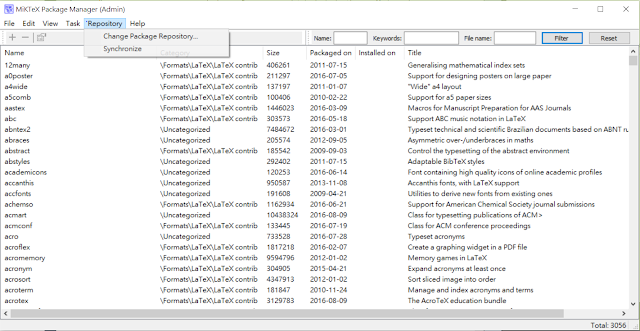## 2016/09/18

### [Windows] Visual Studio + OpenCV

Reference:

Setting Up OpenCV 3.1 in Visual Studio 2015 (Youtube Video)

To make OpenCV work correctly with Visual Studio 2015, you should download OpenCV which version is newer than 3.1

You can use the testing code below to confirm whether it works

#include <iostream>
#include <opencv2/core/core.hpp>
#include <opencv2/highgui/highgui.hpp>

at the beginning of the source code
#include
#include
#include

using namespace std;
using namespace cv;

int main(int argc, char** argv)
{
argv = "D:\\picure.jpg";
Mat image;

if (!image.data)
{
cout << "No Image" << std::endl;
return -1;
}

namedWindow("Display", CV_WINDOW_NORMAL);
imshow("Display", image);
waitKey(0);

return 0;
}


## 2016/08/13

### [LaTex] Windows下的Latex中文環境

由於seminar與論文寫作的需要，得先建構好LaTex的環境，而原本的LaTex是不支援中文字的，需要額外package (package名稱CJK ，代表 Chinese、Japanese、Korean)才行，這篇文章就是紀錄解決LaTex處理中文的問題。

以Windows為例，安裝MikTex (過程可以參考 這則PDF，但是中文字我不是用他提到的方法解決，後面會說)MikText Package Manager

裝好MikTex之後，基本上他會給你一個叫做Texworks的編輯器，不過介面有點陽春

(MikTex跟Texmaker像是compiler跟IDE的關係，意即你可以選擇自己喜歡的editor來用 [註1])

接著打開Texmaker，左邊區域是檔案架構，中間是編輯區，右邊是編譯後預覽PDFTexmaker執行畫面

新增一個文件，並且copy-paste底下取自ptt LaTex版的範例 [註2]
\documentclass{article}

\usepackage{xeCJK}    %打中文必備
\setCJKmainfont{新細明體}     %設定中文字型，而英文不去更動

\begin{document}

document using the article class, $\displaystyle\sum_{i=1}^p L_i \leq n \leq \sum_{i=1}^p U_i$,
with the fontspec package to easily select fonts.
\end{document}
之後點"快速編譯"左邊的箭頭來compile，或者使用快捷鍵F1

裝好回到Texmaker按F1，就能順利compile成功!!

順便附上爬文時找到一些教學介紹

http://web.math.isu.edu.tw/yeh/HowTo/HowToTex/latex123.pdf (雖然是2004的文件，不過應該是基礎入門的經典了，原本網站已經連不上，這份PDF是在義守大學網頁找到的)

XeTeX：解決 LaTeX 惱人的中文字型問題:
http://www.hitripod.com/blog/2011/04/xetex-chinese-font-cjk-latex/

Miktex+texstudio支持中文内容的方法

[註2] https://www.ptt.cc/bbs/LaTeX/M.1366782607.A.202.html
[註3] http://tex.stackexchange.com/questions/319150/miktex-xetex-def-package-installation

[autonumber]

[title]

[ext]

[NUMBER]

## 2016/07/19

### [Software] PDFSAM (split & merge)

Official Site: http://www.pdfsam.org/#

p.s 阿榮福利味有免安裝的版本
http://www.azofreeware.com/2007/11/pdf-split-and-merge-07sr1.html

## 2016/07/11

### [ACM] Q10008

#include
#include <stdio.h>
#include <string.h>
#include <ctype.h>
#include <algorithm>

struct item{
char chr;
int time;
};

using namespace std;

int main(){

int n;
item data;
char str="", line;

for(int i=0; i<26; i++){
data[i].chr = 'A'+i;
data[i].time=0;
}

scanf("%d", &n);
getchar();

while(n){
gets(line);
strcat(str, line);
n--;
}

for(int i=0; i<strlen(str); i++){

if(isalpha(str[i])){

if(isupper(str[i])){
//upper letter
data[str[i]-'A'].time++;

}else{
//lower letter
data[str[i]-'a'].time++;
}
}

}

//sort
for(int i=0; i<26; i++){

for(int j=i+1; j<26; j++){

if(data[j].time > data[i].time){
swap(data[i], data[j]);

}else if(data[i].time == data[j].time){

if(toupper(data[i].chr) > toupper(data[j].chr)){
swap(data[i], data[j]);
}
}
}
}

//display result
for(int i=0; i<26; i++){
if(data[i].time > 0){
printf("%c %d\n", data[i].chr, data[i].time);
}
}

return 0;